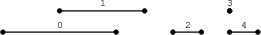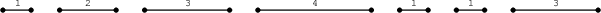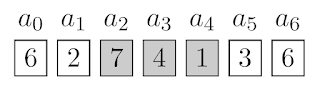## Sunday, March 25, 2018

### Codility - Lesson 16 Greedy algorithms - 2. MaxNonoverlappingSegments

Source Link:
https://app.codility.com/programmers/lessons/16-greedy_algorithms/max_nonoverlapping_segments/

Question:
Located on a line are N segments, numbered from 0 to N − 1, whose positions are given in zero-indexed arrays A and B. For each I (0 ≤ I < N) the position of segment I is from A[I] to B[I] (inclusive). The segments are sorted by their ends, which means that B[K] ≤ B[K + 1] for K such that 0 ≤ K < N − 1.
Two segments I and J, such that I ≠ J, are overlapping if they share at least one common point. In other words, A[I] ≤ A[J] ≤ B[I] or A[J] ≤ A[I] ≤ B[J].
We say that the set of segments is non-overlapping if it contains no two overlapping segments. The goal is to find the size of a non-overlapping set containing the maximal number of segments.
For example, consider arrays A, B such that:
A = 1 B = 5 A = 3 B = 6 A = 7 B = 8 A = 9 B = 9 A = 9 B = 10
The segments are shown in the figure below.The size of a non-overlapping set containing a maximal number of segments is 3. For example, possible sets are {0, 2, 3}, {0, 2, 4}, {1, 2, 3} or {1, 2, 4}. There is no non-overlapping set with four segments.
Write a function:
int solution(int A[], int B[], int N);
that, given two zero-indexed arrays A and B consisting of N integers, returns the size of a non-overlapping set containing a maximal number of segments.
For example, given arrays A, B shown above, the function should return 3, as explained above.
Assume that:
• N is an integer within the range [0..30,000];
• each element of arrays A, B is an integer within the range [0..1,000,000,000];
• A[I] ≤ B[I], for each I (0 ≤ I < N);
• B[K] ≤ B[K + 1], for each K (0 ≤ K < N − 1).
Complexity:
• expected worst-case time complexity is O(N);
• expected worst-case space complexity is O(N), beyond input storage (not counting the storage required for input arguments).

My Solution:

## Sunday, February 25, 2018

### Codility - Lesson 16 Greedy algorithms - 1. TieRopes

Source Link:
https://app.codility.com/programmers/lessons/16-greedy_algorithms/tie_ropes/

Question:
There are N ropes numbered from 0 to N − 1, whose lengths are given in a zero-indexed array A, lying on the floor in a line. For each I (0 ≤ I < N), the length of rope I on the line is A[I].
We say that two ropes I and I + 1 are adjacent. Two adjacent ropes can be tied together with a knot, and the length of the tied rope is the sum of lengths of both ropes. The resulting new rope can then be tied again.
For a given integer K, the goal is to tie the ropes in such a way that the number of ropes whose length is greater than or equal to K is maximal.
For example, consider K = 4 and array A such that:
A = 1 A = 2 A = 3 A = 4 A = 1 A = 1 A = 3
The ropes are shown in the figure below.We can tie:
• rope 1 with rope 2 to produce a rope of length A + A = 5;
• rope 4 with rope 5 with rope 6 to produce a rope of length A + A + A = 5.
After that, there will be three ropes whose lengths are greater than or equal to K = 4. It is not possible to produce four such ropes.
Write a function:
class Solution { public int solution(int K, int[] A); }
that, given an integer K and a non-empty zero-indexed array A of N integers, returns the maximum number of ropes of length greater than or equal to K that can be created.
For example, given K = 4 and array A such that:
A = 1 A = 2 A = 3 A = 4 A = 1 A = 1 A = 3
the function should return 3, as explained above.
Assume that:
• N is an integer within the range [1..100,000];
• K is an integer within the range [1..1,000,000,000];
• each element of array A is an integer within the range [1..1,000,000,000].
Complexity:
• expected worst-case time complexity is O(N);
• expected worst-case space complexity is O(N), beyond input storage (not counting the storage required for input arguments).
My Solution:
Notes:
1. notice that only "adjacent ropes" can be tied
// so, the problem is simple; we can use "greedy" method
2.
int total =0;
int currentLength=0;
3.
if(currentLength >= K){
total++;
currentLength=0; // update
}
4.
return total;

## Sunday, January 28, 2018

### Codility - Lesson 16 Greedy algorithms

Source Link:
https://app.codility.com/programmers/lessons/16-greedy_algorithms/

Some Notes:

Greedy programming is a method by which a solution is determined based on making the locally optimal choice at any given moment. In other words, we choose the best decision from the viewpoint of the current stage of the solution.

Depending on the problem, the greedy method of solving a task may or may not be the best approach. If it is not the best approach, then it often returns a result which is approximately correct but suboptimal.

16.1. The Coin Changing problem

For a given set of denominations, you are asked to find the minimum number of coins with which a given amount of money can be paid. That problem can be approached by a greedy algorithm that always selects the largest denomination not exceeding the remaining amount of money to be paid. As long as the remaining amount is greater than zero, the process is repeated.

t turns out that the greedy algorithm is correct for only some denomination selections, but not for all.
For example, for coins of values 1, 2 and 5 the algorithm returns the optimal number of coins for each amount of money, but for coins of values 1, 3 and 4 the algorithm may return a suboptimal result.

16.1: The greedy algorithm for finding change.
1 def greedyCoinChanging(M, k):
2 n = len(M)
3 result = []
4 for i in xrange(n - 1, -1, -1):
5 result += [(M[i], k // M[i])]
6 k %= M[i]
7 return result

The time complexity of the above algorithm is O(n) as the number of coins is added once for every denomination.

## Saturday, December 30, 2017

### Codility - Lesson 15 Caterpillar Method - 3. CountDistinctSlices

Source Link:

Question:
An integer M and a non-empty zero-indexed array A consisting of N non-negative integers are given. All integers in array A are less than or equal to M.
A pair of integers (P, Q), such that 0 ≤ P ≤ Q < N, is called a slice of array A. The slice consists of the elements A[P], A[P + 1], ..., A[Q]. A distinct slice is a slice consisting of only unique numbers. That is, no individual number occurs more than once in the slice.
For example, consider integer M = 6 and array A such that:
A = 3 A = 4 A = 5 A = 5 A = 2
There are exactly nine distinct slices: (0, 0), (0, 1), (0, 2), (1, 1), (1, 2), (2, 2), (3, 3), (3, 4) and (4, 4).
The goal is to calculate the number of distinct slices.
Write a function:
class Solution { public int solution(int M, int[] A); }
that, given an integer M and a non-empty zero-indexed array A consisting of N integers, returns the number of distinct slices.
If the number of distinct slices is greater than 1,000,000,000, the function should return 1,000,000,000.
For example, given integer M = 6 and array A such that:
A = 3 A = 4 A = 5 A = 5 A = 2
the function should return 9, as explained above.
Assume that:
• N is an integer within the range [1..100,000];
• M is an integer within the range [0..100,000];
• each element of array A is an integer within the range [0..M].
Complexity:
• expected worst-case time complexity is O(N);
• expected worst-case space complexity is O(M), beyond input storage (not counting the storage required for input arguments).
My Solution:
Notes:
1. // main idea: use "boolean[]" to record if an integer is already seen, also use "leftEnd" and "rightEnd"
boolean[] seen = new boolean[M+1]; // from 0 to M
2. // key point: move the "leftEnd" and "rightEnd" of a slice
while(leftEnd < A.length && rightEnd < A.length){
...
}
3. // case 1: distinct (rightEnd)
4. // case 2: not distinct
5.
return numSlice;

### Codility - Lesson 15 Caterpillar Method - 2. CountTriangles

Source Link:
https://app.codility.com/programmers/lessons/15-caterpillar_method/count_triangles/

Question:

A zero-indexed array A consisting of N integers is given. A triplet (P, Q, R) is triangular if it is possible to build a triangle with sides of lengths A[P], A[Q] and A[R]. In other words, triplet (P, Q, R) is triangular if 0 ≤ P < Q < R < N and:
• A[P] + A[Q] > A[R],
• A[Q] + A[R] > A[P],
• A[R] + A[P] > A[Q].
For example, consider array A such that:
A = 10 A = 2 A = 5 A = 1 A = 8 A = 12
There are four triangular triplets that can be constructed from elements of this array, namely (0, 2, 4), (0, 2, 5), (0, 4, 5), and (2, 4, 5).
Write a function:
class Solution { public int solution(int[] A); }
that, given a zero-indexed array A consisting of N integers, returns the number of triangular triplets in this array.
For example, given array A such that:
A = 10 A = 2 A = 5 A = 1 A = 8 A = 12
the function should return 4, as explained above.
Assume that:
• N is an integer within the range [0..1,000];
• each element of array A is an integer within the range [1..1,000,000,000].
Complexity:
• expected worst-case time complexity is O(N2);
• expected worst-case space complexity is O(N), beyond input storage (not counting the storage required for input arguments).
My Solution:
Notes:
1. // important: sort the edges, so that we just need to check if "1st edge + 2nd edge > 3rd edge"
Arrays.sort(A);
2. // Using "Caterpillar method", so we can have O(n^2), not O(n^3)
// key point of "Caterpillar method"
if(rightEnd < A.length && A[i] + A[leftEnd] > A[rightEnd]){
rightEnd++; // increase the Caterpillar
}
else{
numTriangle = numTriangle + (rightEnd - leftEnd - 1);
leftEnd++; // decrease the Caterpillar
}
3.
return numTriangle;

### Codility - Lesson 15 Caterpillar Method - 1. AbsDistinct

Source Link:

Question:
A non-empty zero-indexed array A consisting of N numbers is given. The array is sorted in non-decreasing order. The absolute distinct count of this array is the number of distinct absolute values among the elements of the array.
For example, consider array A such that:
A = -5 A = -3 A = -1 A = 0 A = 3 A = 6
The absolute distinct count of this array is 5, because there are 5 distinct absolute values among the elements of this array, namely 0, 1, 3, 5 and 6.
Write a function:
class Solution { public int solution(int[] A); }
that, given a non-empty zero-indexed array A consisting of N numbers, returns absolute distinct count of array A.
For example, given array A such that:
A = -5 A = -3 A = -1 A = 0 A = 3 A = 6
the function should return 5, as explained above.
Assume that:
• N is an integer within the range [1..100,000];
• each element of array A is an integer within the range [−2,147,483,648..2,147,483,647];
• array A is sorted in non-decreasing order.
Complexity:
• expected worst-case time complexity is O(N);
• expected worst-case space complexity is O(N), beyond input storage (not counting the storage required for input arguments).
My Solution:

Notes:
1. // using "Set"
Set<Integer> set = new HashSet<>();
2. // note: using "Math.abs(int)"
if( set.contains( Math.abs(A[i]) ) == false ){
set.add( Math.abs(A[i]) );
}
3.
return set.size();

### Codility - Lesson 15 Caterpillar Method

Source Link:
https://app.codility.com/programmers/lessons/15-caterpillar_method/

Some Notes:
The Caterpillar method is a likeable name for a popular means of solving algorithmic tasks.  We remember the front and back positions of the caterpillar, and at every step either of them is moved forward.

15.1. Usage example
For example, in the following sequence we are looking for a subsequence whose total equals s = 12.Let’s initially set the caterpillar on the first element. Next we will perform the following steps:

if we can, we move the right end (front) forward and increase the size of the caterpillar;

otherwise, we move the left end (back) forward and decrease the size of the caterpillar.

In this way, for every position of the left end we know the longest caterpillar that covers elements whose total is not greater than s. If there is a subsequence whose total of elements equals s, then there certainly is a moment when the caterpillar covers all its elements.

15.1: Caterpillar in O(n) time complexity.
1 def caterpillarMethod(A, s):
2 n = len(A)
3 front, total = 0, 0
4 for back in xrange(n):
5 while (front < n and total + A[front] <= s):
6 total += A[front]
7 front += 1
8 if total == s:
9 return True
10 total -= A[back]
11 return False

Notice that at every step we move the front or the back of the caterpillar, and their positions will never exceed n. Thus we actually get an O(n) solution.

## Thursday, November 2, 2017

### Codility - Lesson 14 Binary Search Algorithm - 1. MinMaxDivision

Source Link:
https://codility.com/programmers/lessons/14-binary_search_algorithm/min_max_division/

Question:
You are given integers K, M and a non-empty zero-indexed array A consisting of N integers. Every element of the array is not greater than M. You should divide this array into K blocks of consecutive elements. The size of the block is any integer between 0 and N. Every element of the array should belong to some block. The sum of the block from X to Y equals A[X] + A[X + 1] + ... + A[Y]. The sum of empty block equals 0. The large sum is the maximal sum of any block.

For example, you are given integers K = 3, M = 5 and array A such that:
A = 2 A = 1 A = 5 A = 1 A = 2 A = 2 A = 2

The array can be divided, for example, into the following blocks:
• [2, 1, 5, 1, 2, 2, 2], [], [] with a large sum of 15;
• , [1, 5, 1, 2], [2, 2] with a large sum of 9;
• [2, 1, 5], [], [1, 2, 2, 2] with a large sum of 8;
• [2, 1], [5, 1], [2, 2, 2] with a large sum of 6.
The goal is to minimize the large sum. In the above example, 6 is the minimal large sum.

Write a function:
class Solution { public int solution(int K, int M, int[] A); }
that, given integers K, M and a non-empty zero-indexed array A consisting of N integers, returns the minimal large sum.

For example, given K = 3, M = 5 and array A such that:
A = 2 A = 1 A = 5 A = 1 A = 2 A = 2 A = 2
the function should return 6, as explained above.

Assume that:
• N and K are integers within the range [1..100,000];
• M is an integer within the range [0..10,000];
• each element of array A is an integer within the range [0..M].
Complexity:
• expected worst-case time complexity is O(N*log(N+M));
• expected worst-case space complexity is O(1), beyond input storage (not counting the storage required for input arguments).
Elements of input arrays can be modified.

My Solution:
Notes:
1. main idea:
// The goal is to find the "minimal large sum"
// We use "binary search" to find it (so, it can be fast)
// We assume that the "min max Sum" will be
// between "min" and "max", each time we try "mid"
2.
for(int i=0; i<A.length; i++){
maxSum = maxSum + A[i]; // maxSum: sum of all elements
minSum = Math.max(minSum, A[i]); // minSum: at least one max element
}
3. the max one must be an "ok" result
int possibleResult = maxSum;
4. do "binary search" (search for better Sum)
while(minSum <= maxSum){
int midSum = (minSum + maxSum) /2;
boolean ok = checkDivisable(midSum, K, A);
if(ok == true){
possibleResult = midSum;
maxSum = midSum - 1
}
else
minSum = midSum + 1;
}
}
5.
return possibleResult;
6. check if it can be divided by using the minMaxSum = "mid", into K blocks ?
public boolean checkDivisable(int mid, int k, int[] a)

### Codility - Lesson 16 Greedy algorithms - 2. MaxNonoverlappingSegments

Source Link: https://app.codility.com/programmers/lessons/16-greedy_algorithms/max_nonoverlapping_segments/ Question: Located on a line ...